# 1.学习总结

## 1.1树结构思维导图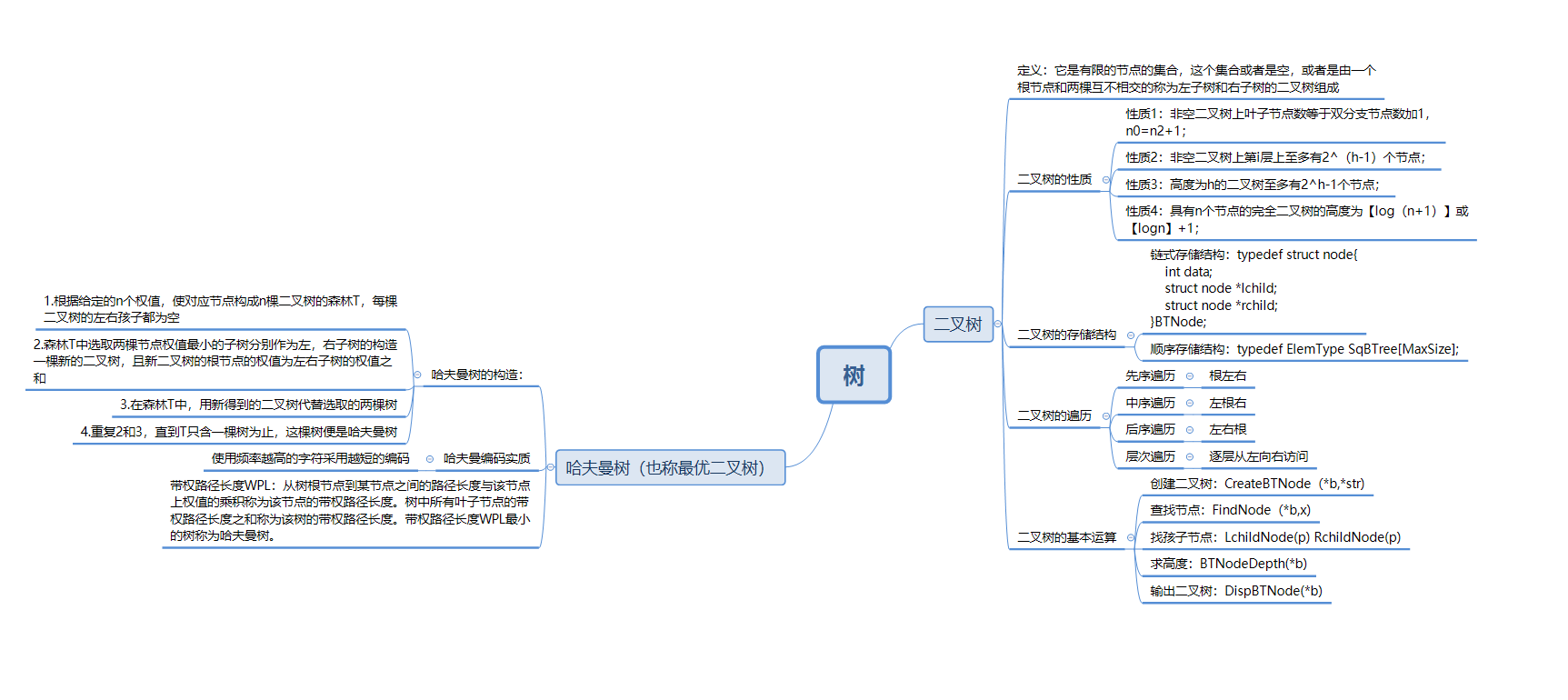# 2.PTA实验作业

## 2.11题目： jmu-ds-表达式树

### 2.12设计思路（伪代码或流程图）

void InitExpTree(BTree &T,string str)

while(表达式未结束)
{
if(str[i]==数字字符) 生成一个只有根节点的子树T。stacktree.push(T)
if(str[i]==运算符)
{
while(str[i]<op栈顶运算符)栈顶优先级别高
{    创建一个树结点T，数据为op.top()
stacktree弹出2个根节点T1,T2
T->lchild=T1;T->rchild=T2;
stacktree.push(T);
}
if(str[i]>op栈顶元素运算符) op.push(str[i])
if(str[i]==op栈顶运算符) op.pop().
}
double EvaluateExTree(BTree T)
{
if(T==NULL) return 0;
if(T->data为数字字符) return T->data-'0'
else{
switch(T->data){
+：return EvaluateExTree(T->lchild) + EvaluateExTree(T->rchild); break；//递归结束标志
-：return EvaluateExTree(T->lchild) - EvaluateExTree(T->rchild); break;  //递归结束标志
*：return EvaluateExTree(T->lchild) * EvaluateExTree(T->rchild); break;  //递归结束标志
/：if((EvaluateExTree(T->rchild)==0)) 输出divide 0 error  exit(0) break;
else return EvaluateExTree(T->lchild) / EvaluateExTree(T->rchild); break;  //递归结束标志
}


### 2.13代码截图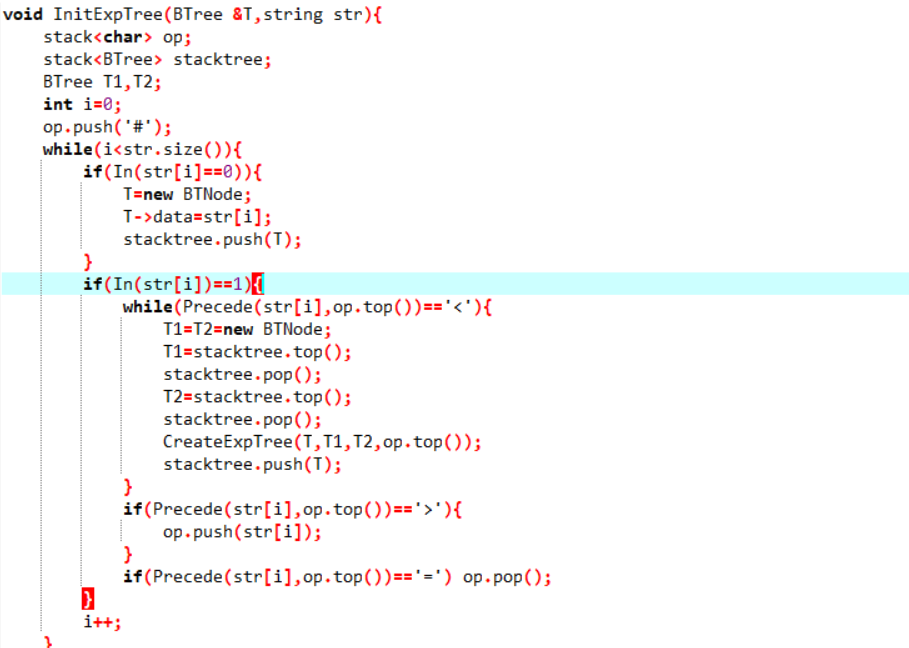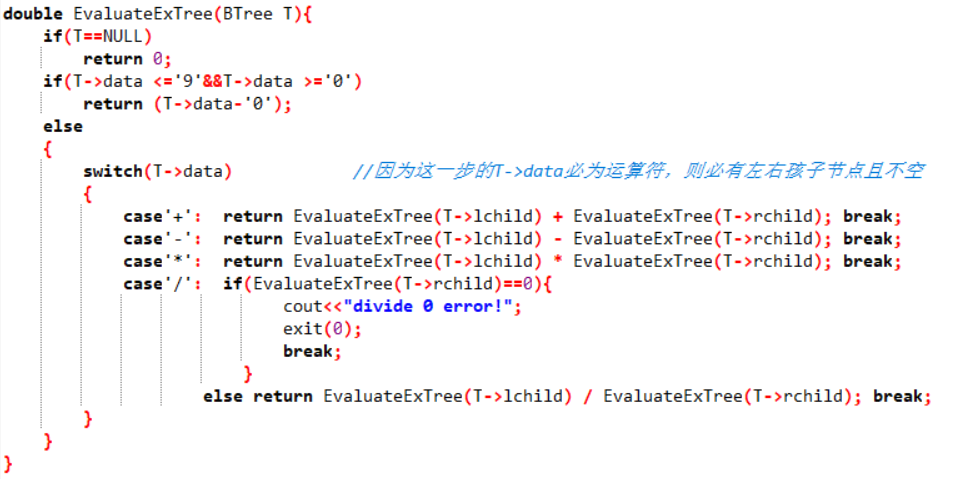### 2.14PTA提交列表说明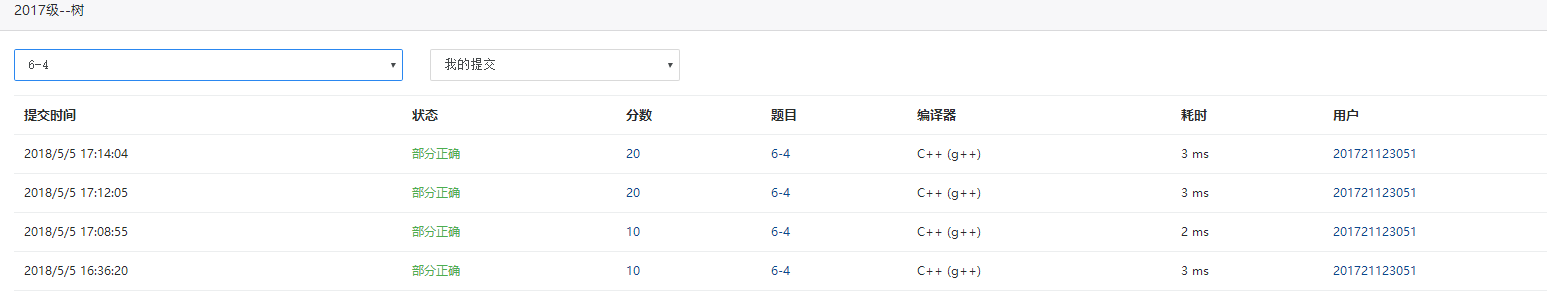#### 分析：表达式树的建立是错误的但是思路应该没有问题，但是还是不够完整，在借用志坚同学的表达式树建立代码后调试求值函数，发现除数为0是会多输出一个0，需要使用exit(0)正常退出，不然求值函数会返回0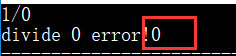## 2.21题目 ：还原二叉树

### 2.22 设计思路（伪代码或流程图）

BTNode*CreateBT(char *pre,char *in,int n)//根据先序和中序建立二叉树
{
若n<=0,返回空指针，递归结束
创建根节点BT,BT->data=*pre;
查找根节点在中序序列的位置k；
创建左子树：BT->lchild=CreateBT(pre+1.in,k)
创建右子树：BT->rchild=CreateBT(pre+k+1,in+k+1,n-k-1)
返回BT
}


### 2.23代码截图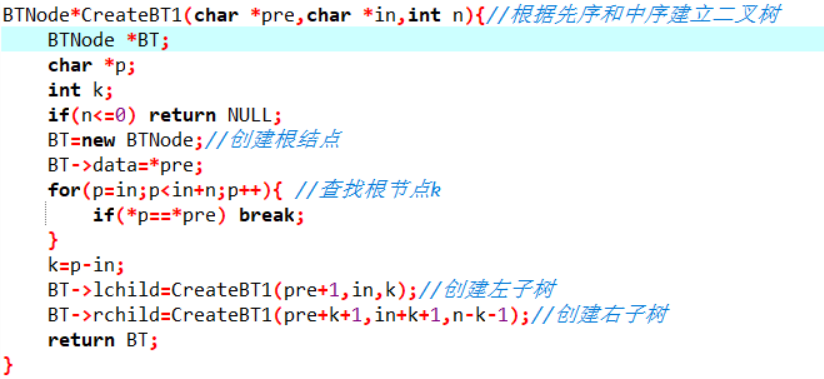### 2.24 PTA提交列表说明#### 分析：虽然该题是一遍过，但是还是有需要注意的地方，就是创建左右子树的递归式的参数，左子树的先序要进一位pre+1，中序序列依旧是原来的in，长度k是中序序列中根节点的位置，右子树的先序是除根节点和左子树剩下的部分所以是pre+k+1，中序是根节点右半部分所以是in+k+1,长度是n-k-1## 2.31题目：二叉树叶子结点带权路径长度和

### 2.32 设计思路（伪代码或流程图）

BTNode *trans(string a,int i,int maxsize)//顺序结构转链式结构
{
if(i>字符串长度maxsize-1||i<=0) return NULL
if(a[i]=='#') return NULL
创建根节点BT，BT->data=a[i]
创建左子树:BT->lchild=trans(a,2*i,maxsize)
创建右子树:BT->rchild=trans(a,2*i+1,maxsize)
返回BT
}
int hfmswpl(BTNode* FBT,int h)//叶子结点带权路径长度和
{
if(FBT为空) return 0 递归结束
esle{
if(FBT是叶子结点)
{
求和:sum=sum+FBT->data*h   (sum为全局变量int型，初值为0)
}
左子树递归:hfmswpl(FBT->lchild,h+1)
右子树递归:hfmswpl(FBT->rchild,h+1)
}


### 2.33代码截图### 2.34 PTA提交列表说明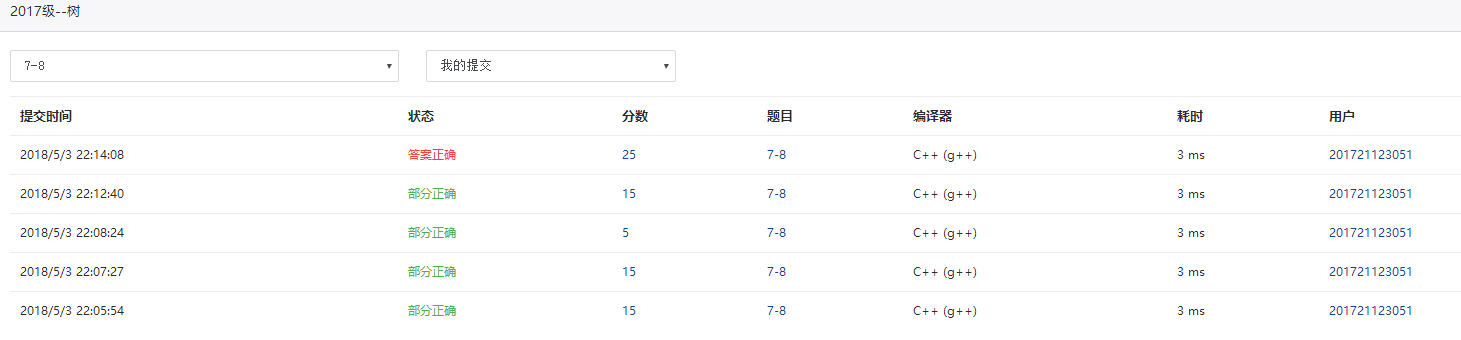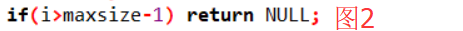# 3.截图本周题目集的PTA最后排名

## 3.1 PTA排名# 4. 阅读代码

void levelnumber(BTNode *b,int h,int a[])
{
if(b==NULL) return ;
else
{
a[h]++;
levelnumber(b->lchild,h+1,a);
levelnumebr(b->rchild,h+1,a);
}
}
int fun(BTNode *b)
{
int width=0,i;
int a[MaxSize];
for(i=1;i<MaxSzie;i++) a[i]=0;
levelnumber(b,1,a);
i=1;
while(a[i]!=0)
{
if(a[i]>width) width=a[i];
i++;
}
return width;
}


# 5. 代码Git提交记录截图posted @ 2018-05-05 19:06  朱杰伟  阅读(299)  评论(4编辑  收藏  举报